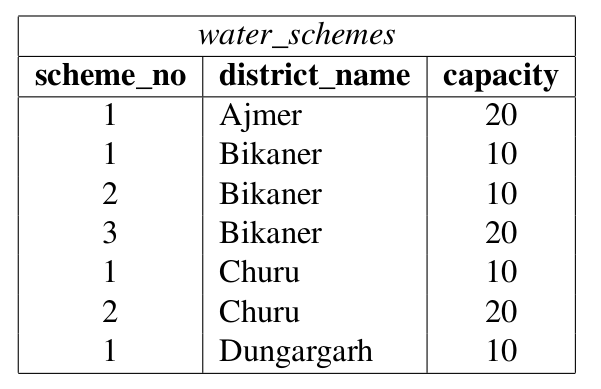# GATE | GATE-CS-2016 (Set 2) | Question 62

• Difficulty Level : Easy
• Last Updated : 28 Jun, 2021

Consider the following database table named water_schemes :Attention reader! Don’t stop learning now.  Practice GATE exam well before the actual exam with the subject-wise and overall quizzes available in GATE Test Series Course.

Learn all GATE CS concepts with Free Live Classes on our youtube channel.

The number of tuples returned by the following SQL query is

```with total(name, capacity) as
select district_name, sum(capacity)
from water_schemes
group by district_name
with total_avg(capacity) as
select avg(capacity)
from total
select name
from total, total_avg
where total.capacity >= total_avg.capacity```

(A) 1
(B) 2
(C) 3
(D) 4

Explanation: First group by district name is performed and total capacities obtained as following

```   Ajmer 20
Bikaner 40
Charu 30
Dungargarh 10 ```

Then average capacity is computed,

```Average Capacity = (20 + 40 + 30 + 10)/4
= 100/4
= 25.
```

Finally districts with more than average are selected.

```Bikaner is 40 which is greater than average (25)
Charu is 30 which is also greater than average (25).